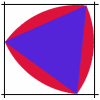#### You may also like### Is There a Theorem?

Draw a square. A second square of the same size slides around the first always maintaining contact and keeping the same orientation. How far does the dot travel?### The Old Goats

A rectangular field has two posts with a ring on top of each post. There are two quarrelsome goats and plenty of ropes which you can tie to their collars. How can you secure them so they can't fight each other but can reach every corner of the field?### Rolling Triangle

The triangle ABC is equilateral. The arc AB has centre C, the arc BC has centre A and the arc CA has centre B. Explain how and why this shape can roll along between two parallel tracks.

# Turning Triangles

##### Age 11 to 14 Challenge Level:
Lorna from Sutton High School sent us her work on this problem. Well done, Lorna!

When a triangle is rolled along a horizontal line (the baseline), the paths of the vertices can be described as below.

Equilateral triangle ABC - The overall pattern of paths forms three overlapping arcs, the radii of which are the triangle side's length. The arcs all rest upon the same horizontal line, and each arc starts a triangle side's length away from the one before. The paths intersect after the vertices concerned have both travelled 120º.

Right angled isosceles triangle ABC - The overall pattern of paths this time consists of two different sized arcs - small arcs (radius of equal sides AB and AC) and big arcs (radius of side BC). The path of vertex A only ever forms small arcs, the vertex being formed by the two equal short sides. Vertices B and C form a pattern of arcs first big, then small, then big etc., because these vertices are both connected to one long and one short side. No two paths ever follow the same track.

Right angled scalene triangle ABC - This time the pattern consists of three different sized arcs, each the radius of a different triangle side. Each path consists of two arc sizes (either big and medium, big and small or medium and small) because each vertex is made from the joining of two sides of different lengths.

Overall Explanation - When a vertex reaches the baseline, it becomes the pivot that the triangle rotates around. Therefore it stays on the baseline for one rotation. Because there are three sides, each vertex first reaches the baseline every third rotation, but being a pivot means that it does not make a path. This is why each path is made up of a pattern of only two different arcs.